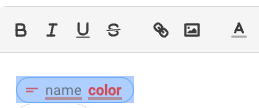# If statement as a formula

I'm creating a snippet that will use the same if statement in multiple locations, but with different values for what it's comparing

Is it possible to store an if statement in a variable and treat it like a formula?

It will be triggering one of multiple imports based on a dropdown menu

Also, I've found an issue with rich text formatting, coloured text inside a formula loses colour data when inserted

{test=(x) -> "▐███▌" if lower(x)="red" else "" }
{=test("Red")}

Unfortunately can't do colours in here, but imagine the squares are red, the output would still be unformatted

Hi @chris.d Welcome to the forum!Just so that I understand, are you looking for something like this:

{func1=()->"Hello from func1"}{func2=()->"Hello from func2"}
{formtoggle: name=use_1;default=yes}{endformtoggle}
{func_to_use=func1 if use_1 else func2}{=func_to_use()}

You can define a function like this, and then pass it additional values as arguments. If you give more details, I can give you a better example snippet for this.

Here's one way to do this:

``````Select an import: {formmenu: Import 1; Import 2; Import 3; name=selected_import}

{if: `selected_import` = "Import 1"}
{import: Import 1}
{elseif: `selected_import` = "Import 2"}
{import: Import 2}
{elseif: `selected_import` = "Import 3"}
{import: Import 3}
{endif}
``````

Please replace "Import 1", "Import 2", and "Import 3" with the actual names of the imports you want to trigger.

Let me know if it works for you.

1 Like

You can format the whole cell red, by selecting it and then choosing the red color, like so:If you give more details about how exactly you're trying to color this text, then I can help you better.